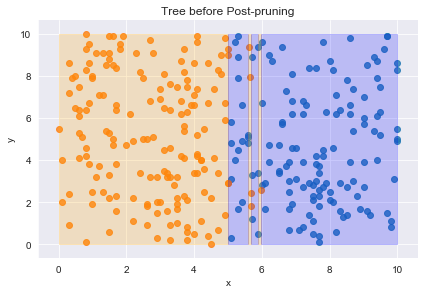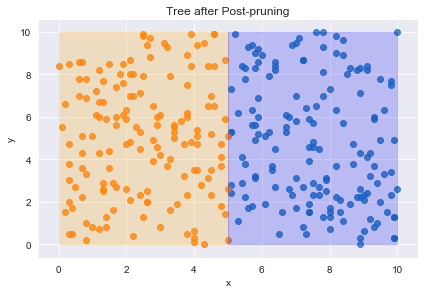In :
import numpy as np
import pandas as pd

from pprint import pprint

from decision_tree_functions import decision_tree_algorithm, make_predictions, calculate_accuracy
from helper_functions import generate_data, create_plot, train_test_split


# 1. Post-Pruning¶

In :
def filter_df(df, question):
feature, _, value = question.split()
df_yes = df[df[feature] <= float(value)]
df_no  = df[df[feature] >  float(value)]

return df_yes, df_no

In :
def pruning_result(tree, df_train, df_val):

leaf = df_train.label.value_counts().index
errors_leaf = sum(df_val.label != leaf)
errors_decision_node = sum(df_val.label != make_predictions(df_val, tree))

if errors_leaf <= errors_decision_node:
return leaf
else:
return tree

In :
tree = {'x <= 5.0': [{"y <= 5": [True,
False]},
False]}

In :
def post_pruning(tree, df_train, df_val):

question = list(tree.keys())

# base case
return pruning_result(tree, df_train, df_val)

# recursive part
else:
df_train_yes, df_train_no = filter_df(df_train, question)
df_val_yes, df_val_no = filter_df(df_val, question)

return pruning_result(tree, df_train, df_val)

In :
# np.random.seed(0)
df_train = generate_data(n=300, n_random_outliers=5)